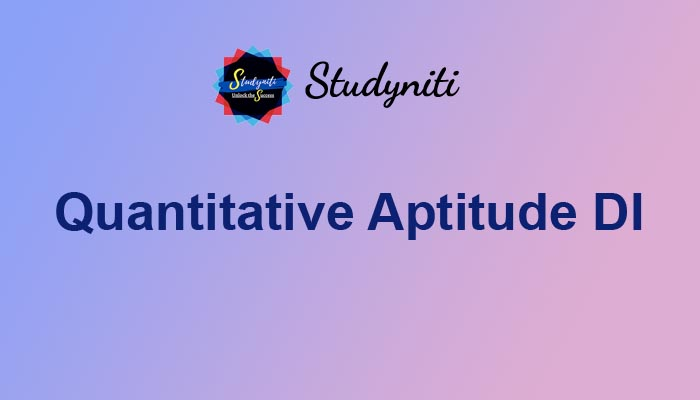## Payment Processing...# Quantitative Aptitude DI - Oct 10 2020

Here we are providing new series of Quantitative Aptitude Questions for upcoming exams, so the aspirants can practice it on a daily basis.

On the festive season Cabs can be booked from five different cab services uber, Ola, mehru, spice cabs, and easy cabs at a cheap rate and booking time start at 12 noon to 4:00 pm of the same day for local travelling.

Fare of every cab is flexible and changes by time, the graph given below shows the fare of cab when booking starts, fare when booking closes, highest and lowest fare between that period of those five cabs respectively.Ceiling of the rectangle shows the closing fare of cab while floor of the rectangle shows the opening fare of cab.

Ceiling of the line shows highest fare of cab while floor of the lines shows the lower fare of cab. If the price of fare is more than or equal to 300, then 20 % discount is given and when the price of cab is less  than 300 then 10 % discount is given.

1) Praveen books his cab from Uber when its booking starts and books Ola at the time of return when its fare is highest, then what is the ratio of total amount of fare from Uber to that of Ola?

a) 9:16

b) 25:36

c) 2:3

d) 5:7

e) None of these

Answer .A

2) If total amount of fare collected by Ola when booking is about to closed is Rs 32400  and total amount collected by Mehru when booking just started is Rs 18000 , then what is the ratio of total passengers who booked from Ola to that of Mehru?

a) 9:16

b) 25:36

c) 9:10

d) 5:7

e) None of these

3) If a group of  20 people book the cab from Mehru when its booking is about to close, then what is the total amount saved by that group if they booked from Uber when its booking starts instead of booking their cab from Mehru?

a) 1000

b) 1100

c) 1200

d) 1300

e) None of these

4) If total passenger booked cab from  Spice cabs when its price highest is 10 less than the number of passengers booked cab from same agency when its price lowest and ratio between the total fare amount collected by Spice cabs when its price are highest  to that when lowest is 72:117 , then how many passengers booked their cab when price is lowest?

a) 16

b) 36

c) 23

d) 13

e) None of these

5) Cab fare of Taxi4sure when booking starts is the average of the opening & closing fare of Easy cabs and lowest fares of Taxi4sure is 60% of its fare when booking starts. If total fare amount collected by Taxi4sure of 16 passengers when its price is lowest is same as total fare amount collected by Taxi4sure of 10 passengers when its price is highest, then what is the highest fare (without discount ) of Taxi4sure?

a) 916

b) 253

c) 845

d) 450

e) None of these

Answers :

1) Answer: A

Fare from Uber when booking starts = Rs 250

Total fare after 10% discount from uber when booking starts = 250 × 90/100 = Rs 225

Fare from ola when price is highest = Rs 500

Total fare after 20% discount from Ola when price is highest = 500× 80 /100 =Rs 400

Required ratio = 225: 400 = 9: 16

2) Answer: C

Total fare collected by Ola= y × 80% of 450 = 32400

= > y = 90

Total face collected by Mehru = z × 90% of 200 = 18000

= > z = 100

Required ratio = 90: 100 = 9:10

3) Answer: B

Booking from Uber when booking just start = Rs 250

Total fare of 20 people from Uber =20× 90% of 250 = 4500

Booking from Mehru when booking just start = Rs 350

Total fare of 20 people from Mehru = 20 × 80% of 350 = 5600

Savings = 5600 – 4500 = Rs 1100

4) Answer D.

Let the number of passengers that booked their cab when price is highest and when the price is lowest is ‘m’ and ‘m+10 ‘

Booking from Spice cabs when price is highest = Rs 600

Total fare amount of ‘m’ passengers = m × 80% of 600 = 480m

Cab booked from Spice cabs when price is lowest = Rs 200

Total fare amount of ‘m + 10’ passengers = (m + 10) × 90% of 200 = 180(m+10)

Now according to question

480m:180(m+10) = 72:117

m= 3

Number of passenger booked their cab when price is lowest = 10 + 3 = 13

5) Answer: D.

Cab fare of Taxi4sure when booking starts = (150 + 600)/2 = Rs 375

Lowest fare of Taxi4sure = 60% of 375 = Rs225

Total fare amount of 16 passengers when price is lowest = 225 × 16 = Rs 3600

Total fare amount of 10 passengers when price is highest = 10× y = 3600

y = 360

Highest fare of Taxi4sure without discount = 360 × (100/80) = Rs450